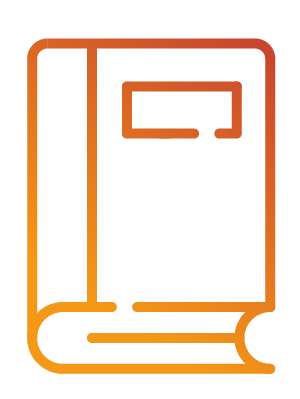# Structural Proof Theory

### Monographie• Pages : XVII-257
•
• Support : Print
• Format : 24 cm.
• Langues : Anglais
• Édition : Original
• Ville : Cambridge
•
• ISBN : 0521793076 (hbk.)
• URL : Lien externe
•
• Date de création : 22-03-2012
• Dernière mise à jour : 22-03-2012

### Résumé

#### Anglais

Structural proof theory is a branch of logic that studies the general structure and properties of logical and mathematical proofs. This book is both a concise introduction to the central results and methods of structural proof theory, and a work of research that will be of interest to specialists. The book is designed to be used by students of philosophy, mathematics and computer science. The book contains a wealth of results on proof-theoretical systems, including extensions of such systems from logic to mathematics, and on the connection between the two main forms of structural proof theory - natural deduction and sequent calculus. The authors emphasize the computational content of logical results. – Contents : – Introduction. – 1. From natural deduction to sequent calculus; – 2. Sequent calculus for institutionistic logic; – 3. Sequent calculus for classical logic; – 4. The quantifiers; – 5. Variants of sequent calculi; – 6. Structural proof analysis of axiomatic theories; – 7. Intermediate logical systems; – 8. Back to natural deduction. – Conclusion: diversity and unity in structural proof theory. – Appendix A. Simple type theory and categorical grammar; – Appendix B. Proof theory and constructive type theory; – Appendix C. A proof editor for sequent calculus [By Aarne Ranta]. – Includes bibliographical references (p. 245-249) and indexes.